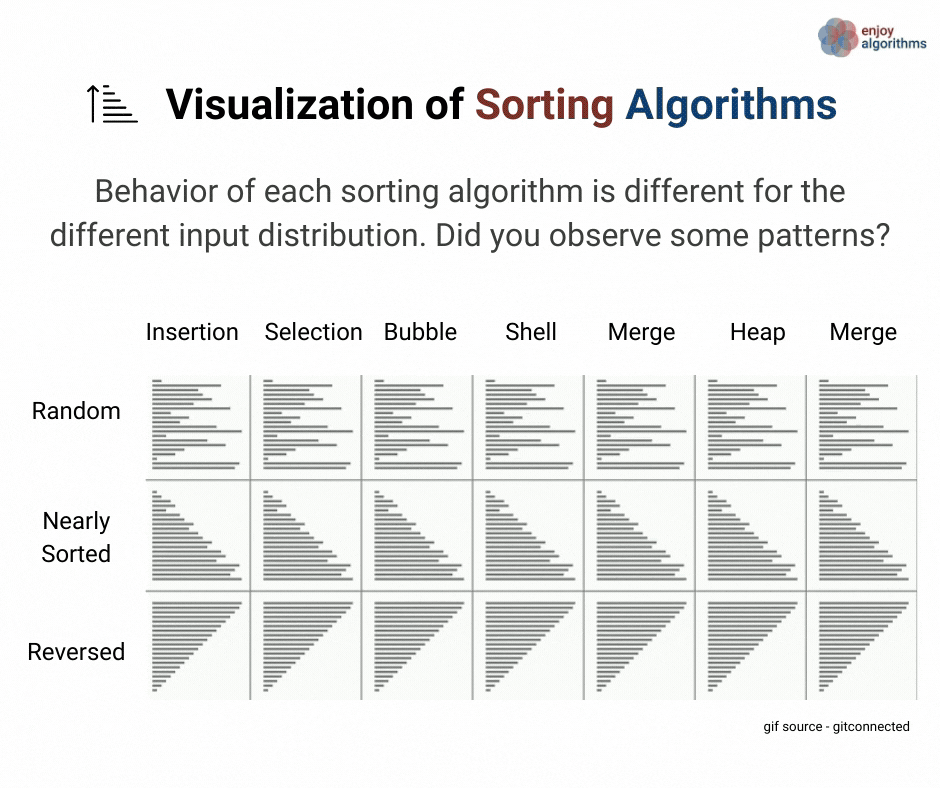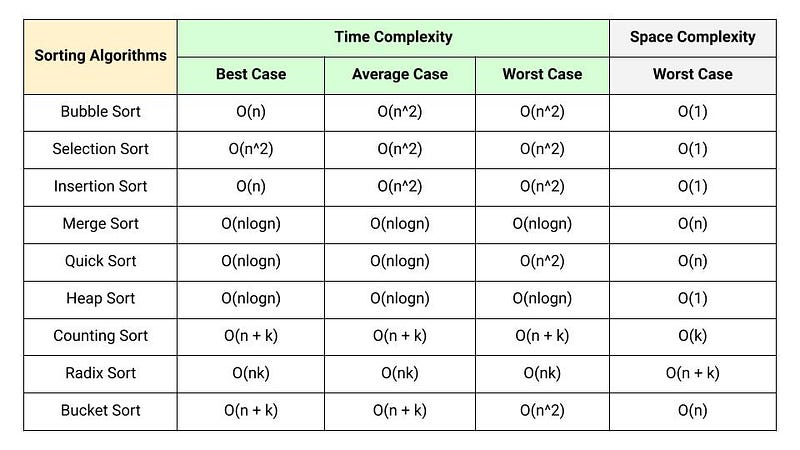# Sorting Algorithms Comparison

Why do we study the design, code, and analysis of the sorting algorithms? Here are some critical reasons:

• We can learn several problem-solving approaches using sorting algorithms: incremental approach (selection and insertion sort), divide and conquer (merge and quick sort), two pointers approach (merging and partition), problem-solving using data structures (heap and tree sort), etc.
• It is one of the best ideas to understand the complexity analysis of recursive and iterative code.
• We also use sorting as a problem-solving approach, i.e., we can solve several problems efficiently by organizing the data into sorted order.

This blog will analyze and compare various sorting algorithms based on different parameters like time complexity, space complexity, in-place, stability, online vs. offline, problem-solving approaches, etc.

### Comparison based on efficiency

Comparison based sorting algorithms

In comparison-based sorting algorithms, we compare elements to determine the order of elements in the final sorted output. All comparison-based sorting algorithms have a complexity lower bound of nlogn. (Think!) We have already seen that any comparison-based sorting algorithm must take O(n log n) time to sort an array of n elements in the worst case.

• Bubble sort: compare elements to place the max elements to the end positions.
• Selection sort: compare elements to place the minimum elements to the front positions.
• Insertion sort: compare elements to decide the position of an element in the partially sorted array.
• Merge sort: compare elements of two sorted elements to merge them into the final sorted array.
• Quicksort: compare elements of partition the unsorted array two different halves around the pivot value.
• Heapsort: compare elements during the heapify process to place the minimum elements to the front of the array (If we are using the min-heap).

As we have seen, the worst-case time complexities of the above sorting algorithms can be categorized into two parts: O(n^2) and O(nlogn).

• O(n^2) sorting algorithms: bubble sort, selection sort, and insertion sort
• O(nlogn) sorting algorithms: merge sort, quicksort, heapsort. Note: The worst-case performance of quicksort is O(n^2), but it works very fast in O(nlogn) time complexity on average. Actually, the chances of occurring the worst-case in very low when all input possibilities are equally likely.

In addition to the comparison operations, we also perform other types of operations in these sorting algorithms. But the count of these operations would always be less than the count of comparison operations. That's why comparison operation is the deciding factor of the time complexity.

• Bubble sort: swapping
• Selection sort: swapping
• Insertion sort: sifting
• Merge sort: allocation of extra memory and data copy
• Quicksort: swapping
• Heapsort: swappingLiner time sorting algorithm

There are sorting algorithms that run faster than O(nlogn) time complexity, but they require special assumptions about the input sequence to determine the sorted order of elements. These sorting algorithms use operations other than comparisons to determine the sorted order and work in O(n) time complexity. So, O(nlogn) lower bound does not apply to these sorting algorithms.

Examples of sorting algorithms that run in linear time are counting sort, radix sort, bucket sort, etc. Counting sort and radix sort assume that the input consists of integers in a small range. At the same time, bucket sort assumes that the input is generated by a random process that distributes elements uniformly over the interval.

Special conditions with linear time sorting algorithms

• Counting sort: each input element is an integer in the range 0 to k.
• Radix sort: Given n integers where each integer can take on up to k possible values.
• Bucket sort: Input is generated by the random process that distributes elements uniformly and independently over the interval [0, 1).

Here is the comparison of time and space complexities of some popular sorting algorithms:### In-place sorting algorithms

A sorting algorithm is In-place if it does not use extra space to manipulate the input but may require a small though nonconstant extra space for its operation. Or we can say a sorting algorithm sorts in place if only a constant number of input array elements are ever stored outside the array.

• In-place sorting algorithms: bubble sort, selection sort, insertion sort, quicksort, heapsort
• Sorting algorithms with extra space: merge sort, counting sort

### Stable sorting algorithms

A sorting algorithm is stable if it does not change the order of elements with the same value.

• Stable sorting algorithms: bubble sort, insertion sort, merge sort.
• Non-stable sorting algorithms: selection sort, quicksort, heapsort, counting sort.
• Stable sorting algorithms work according to this rule: if two items compare as equal, then their relative order will be preserved, i.e., if one comes before the other in the input, it will arrive before the other in the output.
• Stability is essential to preserve order over multiple sorts on the same data set.
• Stability is also not an issue if all keys are different.
• Unstable sorting algorithms can be specially implemented to be stable. One way of doing this is to extend the comparison operation so that comparisons between two data objects with equal keys are decided using the order of the entries in the original input data as a tie-breaker. But remembering this order, however, may require additional time and space.

### Online and offline sorting algorithms

The algorithm that accepts a new element while the sorting process is going on is called the online sorting algorithm. An online algorithm can process its input piece-by-piece in a serial order. In other words, online sorting algorithms can sort the data not without having the entire input available from the beginning.

For example, insertion sort considers one input element per iteration and produces a partially sorted solution without considering future elements. So maintaining a sorted list in the insertion sort, we can place each input item in its proper place as we receive the input. So insertion sort is an online sorting algorithm.

In contrast, an offline algorithm needs the complete input data in the memory from the beginning, or it requires all items to be in memory before sorting can begin. For example, The selection sort algorithm sorts an array by repeatedly finding the minimum element from the unsorted part and putting it at the beginning. Which always requires access to the entire input, so it is an offline algorithm.

### Comparison based on problem-solving approaches

• Divide and conquer approach: merge sort and quick sort
• An incremental approach using nested loops: bucket sort, selection sort, insertion sort
• Problem-solving using data structures: heap sort, tree sort
• Problem-solving using hashing: counting sort

### Critical ideas to think!

• Understanding the sorting algorithms are the best way to learn problem-solving and complexity analysis in the algorithms. In some cases, We often use sorting as a key routine to solve several coding problems.
• Like insertion sort, quick sort has tight code, and the hidden constant factor in its running time is small.
• The insertion sort can be preferred when the input array is almost sorted or small input size.
• Insertion sort is efficient than the selection and bubble sort algorithm.
• Knowing which algorithm is best possible depends heavily on the details of the application and implementation. Quicksort is a popular algorithm for sorting large input arrays in most practical situations because its expected running time is O(nlogn). It also outperforms heap sort in practice.
• Merge sort is the best choice for sorting a linked list. It is relatively easy to implement a merge sort in this situation to require only O(1) extra space. On the other hand, a linked list's slow random-access performance makes some other algorithms, such as quicksort, perform poorly and others like heap sort completely impossible.
• We can parallelize the merge sort's implementation due to the divide-and-conquer method, where every smaller sub-problem is independent of each other.
• If stability is essential and space is available, the merge sort might be the best choice for the implementation.
• How can we improve the running time of quicksort by taking advantage of the fast running time of insertion sort when its input is almost sorted?
• How can we modify the quick sort algorithm to make it stable?
• How can we implement quicksort and merge sort iteratively using stack?
• Some additional sorting algorithms to explore: Shell sort, Tree sort, and Tournament sort

### Critical concepts and problems to explore

Enjoy learning, Enjoy sorting!

Get well-designed application and interview centirc content on ds-algorithms, machine learning, system design and oops. Content will be delivered weekly.